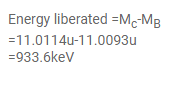# The masses of

Question:

The masses of ${ }^{11} \mathrm{C}$ and ${ }^{11} \mathrm{~B}$ are respectively $11.0114 \mathrm{u}$ and $11.0093 \mathrm{u}$. Find the maximum energy a positron can have in the $\beta^{+}$-decay of ${ }^{11} \mathrm{C}$ to ${ }^{11} \mathrm{~B}$.

Solution: